# Coordinate-wise descent method

(diff) ← Older revision | Latest revision (diff) | Newer revision → (diff)

One of the methods for minimizing a function of several variables based only on the values of the function to be minimized. The method is used when the function is not differentiable or if a calculation of the derivatives involves a large amount of computation. Below the use of the coordinate-wise descent method for minimizing a functionon a setwhere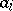and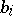are given numbers,, is described and the cases where all or some of the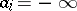or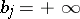are not excluded. Letbe the coordinate vector in which the-th coordinate is equal to 1 and the other coordinates are equal to zero. One specifies an initial approximation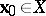,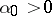. Assume that the-th approximation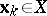is known and that, for some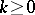. Take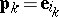, where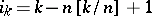andis the integer part of. Then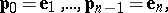i.e. one performs a cyclic selection of the coordinate vectors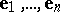. First one checks if the conditions(1)

are fulfilled. If (1) is fulfilled, one sets,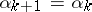. If on the other hand (1) is not fulfilled, one checks the condition(2)

If (2) is fulfilled, one sets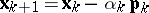,. If conditions (1) and (2) are both not fulfilled, one sets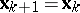,(3)

whereis the parameter of the method,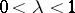. Condition (3) means that if at least one of the conditions (1) and (2) is fulfilled in a single cycle ofiterations involving a selection of all coordinate vectorswith step, then the length of the step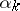is not reduced and is retained at least during the following cycle ofiterations; if on the other hand neither (1) nor (2) is ever fulfilled in the subsequentiterations, the stepis reduced.

Let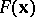be convex and continuously differentiable on, let the set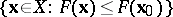be bounded and letbe a positive number. Then the methods (1)–(3) converge, i.e.and the sequence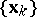converges to the set of minima forin. Ifis not differentiable on, the method need not converge , .

How to Cite This Entry:
Coordinate-wise descent method. F.P. Vasil'ev (originator), Encyclopedia of Mathematics. URL: http://encyclopediaofmath.org/index.php?title=Coordinate-wise_descent_method&oldid=18950
This text originally appeared in Encyclopedia of Mathematics - ISBN 1402006098